# SAS/STAT Examples

## Bayesian Unidimensional IRT Models: Graded Response Model

Contents | SAS Program | PDF

## Overview

This SAS Web Example demonstrates how to fit graded response models by using the MCMC procedure. The graded response model is used to model ordered polytomous data. The Analysis section presents a brief mathematical description of the model. The Example section analyzes an instrument by using the MCMC procedure. Initially, the PROC MCMC model specification is written with prior knowledge of both the number of items and the number of categories per item. This prior knowledge is hard-coded into the PROC MCMC model specification. In other words, the PROC MCMC model specification is written such that the program can be used only for instruments with a specific number of items and for items with a specific number of categories. The purpose of the initial example is to illustrate the basic anatomy of a graded response model as specified in PROC MCMC. The example is then extended to demonstrate how you can use the SAS macro language to generalize the PROC MCMC model specification so that you can reuse your SAS program for instruments that contain any number of items and any number of categories per item. As a result, what begins as a lengthy model specification is reduced to just a few lines of SAS code.

## Analysis

In unidimensional item response theory (IRT) models, an instrument (test) consists of a number of items (questions) that require responses that are to be chosen from a predetermined number of categories (options). The purpose of the instrument is to measure a single latent trait of the test subjects. The latent trait is assumed to be measurable and to have a range that encompasses the real line. An individual’s location within this range, , is assumed to be a continuous random variable. When there are only two response categories, you can use binary response models to analyze the data. See the web example "Bayesian IRT Models: Unidimensional Binary Models" for a discussion of these models and how to implement them by using PROC MCMC. When there are more than two categories and the categories are ordered, meaning that some responses indicate more (or less) of the latent trait being measured, you can use an extension of the binary models known as a graded response model to analyze the data. The purpose of the graded response model is to enable you to estimate the probability that a test subject will choose a particular response for each item, to estimate the levels of the latent traits of the test subjects, and to evaluate how well the items, individually and collectively, measure the test subject’s latent trait.

The graded response model specifies the cumulative probability of scoring in, or selecting, each of K categories or higher as

where is the latent trait, is the discrimination parameter for item j, and is the category boundary location for the kth category of item j. By definition, the probability of responding in the lowest category or higher is 1, and the probability of responding in category K+1 or higher is 0. A plot of the graded response model’s cumulative probabilities as a function of , often referred to as a category boundary curve (CBC), has the shape of an ogive. The point of inflection of a category boundary curve is located at , and the probability of obtaining a category score k or higher is 0.50 at . The slopes of the boundary curves at are proportional to .

If you are familiar with item response theory models for binary responses, you will undoubtedly recognize that the equation for the graded response model’s cumulative probability is identical to the equation for the marginal probability from a two-parameter logistic (2PL) model. In fact, you can think of the graded response model as the successive application of the 2PL model to an ordered series of bifurcated responses (De Ayala 2009, chapter 7).

To compute the marginal probability, , of selecting the kth category of item j, you take the difference between the cumulative probabilities for adjacent categories:

A plot of as a function of is known as an option response function (ORF).

After you fit a graded response model, you can use the parameter estimates to compute the amount of information that is provided by each response category. The option information function (OIF) for each graded response option is the negative of the expected value of the second derivative of the log-likelihood function and is computed as follows:

An item’s information function is the sum of the option information functions:

Similarly, the instrument’s total information function is the sum of the item information functions:

### Bayesian Estimation

Bayesian estimation requires that you specify the likelihood function of the response variable and specify prior distributions for the unknown model parameters. The likelihood for the graded response model is just the probability distribution function of a categorical distribution. To specify the likelihood in PROC MCMC, you use a MODEL statement and the table distribution.

The unknown parameters are , , and . Unless you have specific prior information about these distributions, it is common practice to specify a standard normal distribution for and diffuse prior distributions for the and parameters. In this example, is treated as a random effect that is indexed by test subject, and it is assigned a standard normal prior distribution. The and parameters have theoretical ranges that encompass the real line. It is common practice to assign diffuse normal, truncated normal, or lognormal distributions to the parameters. For each of the J items, the parameters must satisfy the following order constraint: . There are several strategies that you can use to impose these order constraints on the prior distributions. In the example that follows, the order constraints are imposed by specifying truncated normal distributions as the priors, with being specified as the lower truncation boundary for the prior distribution of .

 There are other models for polytomous data besides the graded response model, such as the partial credit model and the generalized partial credit model.

 Category boundary curves are also referred to in the item response theory literature as cumulative probability curves, category characteristic curves, or boundary characteristic curves (De Ayala 2009, chapter 7).

 Option response functions are also referred to in the item response theory literature as category probability curves, category response functions, operating characteristic curves, or option characteristic curves (De Ayala 2009, chapter 7).

## Example

This example fits a graded response model to a hypothetical instrument that has three items. The first item has three categories, the second item has four categories, and the third item has five categories. The following DATA step reads the data set `Graded`. The variables `Item1`, `Item2`, and `Item3` record the responses to the three items on the instrument, and the variable `Person` indexes the test subjects.

```data graded;
input person item1 item2 item3 @@;
datalines;
1 3 2 2 2 1 1 2 3 2 2 3 4 2 2 4 5 2 2 2 6 3 2 3 7 3 2 2 8 3 2 2
9 3 2 2 10 1 2 2 11 2 3 3 12 2 2 2 13 2 1 2 14 2 2 2 15 2 2 3 16 2 3 3
17 3 2 3 18 2 1 2 19 1 2 2 20 2 1 3 21 2 3 3 22 1 1 2 23 2 1 2 24 3 2 3
25 2 2 3 26 2 2 3 27 3 2 2 28 3 2 4 29 2 3 2 30 2 1 2 31 3 2 3 32 3 2 3
33 2 2 2 34 1 1 2 35 2 2 3 36 2 3 3 37 2 2 4 38 1 1 1 39 3 3 3 40 2 2 4

... more lines ...

977 2 2 3 978 2 2 2 979 2 2 3 980 2 3 3 981 1 1 1 982 3 3 4 983 3 2 2 984 2 2 2
985 2 2 2 986 3 3 3 987 2 2 2 988 3 3 4 989 2 2 2 990 3 2 3 991 3 3 3 992 3 3 4
993 2 1 2 994 3 1 2 995 1 2 2 996 2 2 2 997 3 3 3 998 2 2 3 999 2 2 3 1000 3 2 4
;
```

The following six elements are essential to a PROC MCMC specification for a graded response model:

• PROC MCMC statement

• RANDOM statement for

• PARMS statements for and

• PRIOR statements for and

• programming statements that compute the cumulative and marginal probabilities

• MODEL statements for each item

The model specification in PROC MCMC is highly dependent on the number of items contained in the instrument and the number of categories per item. The following statements specify the graded response model for the `Graded` data set:

```ods graphics on;
ods output PostSumInt=PostSumInt;
proc mcmc data=graded nmc=80000 outpost=outpost seed=10000 nthreads=-1;
random theta~normal(0, var=1) subject=person nooutpost;
parms alpha1 1 alpha2 1 alpha3 1;
parms delta12 -1 delta13   1;
parms delta22 -1 delta23   0 delta24  1;
parms delta32 -1 delta33 -.5 delta34 .5 delta35 1;
prior alpha: ~normal(1, var=12);
prior delta12: ~normal(0, var=12);
prior delta13: ~normal(0, var=12, lower=delta12);
prior delta22: ~normal(0, var=12);
prior delta23: ~normal(0, var=12, lower=delta22);
prior delta24: ~normal(0, var=12, lower=delta23);
prior delta32: ~normal(0, var=12);
prior delta33: ~normal(0, var=12, lower=delta32);
prior delta34: ~normal(0, var=12, lower=delta33);
prior delta35: ~normal(0, var=12, lower=delta34);
array cp1; array cp2; array cp3;
array p1; array p2; array p3;
cp1=1;
cp1=logistic(alpha1*(theta-delta12));
cp1=logistic(alpha1*(theta-delta13));
cp2=1;
cp2=logistic(alpha2*(theta-delta22));
cp2=logistic(alpha2*(theta-delta23));
cp2=logistic(alpha2*(theta-delta24));
cp3=1;
cp3=logistic(alpha3*(theta-delta32));
cp3=logistic(alpha3*(theta-delta33));
cp3=logistic(alpha3*(theta-delta34));
cp3=logistic(alpha3*(theta-delta35));
p1=1-cp1;
p1=cp1-cp1;
p1=cp1;
p2=1-cp2;
p2=cp2-cp2;
p2=cp2-cp2;
p2=cp2;
p3=1-cp3;
p3=cp3-cp3;
p3=cp3-cp3;
p3=cp3-cp3;
p3=cp3;
model item1 ~ table(p1);
model item2 ~ table(p2);
model item3 ~ table(p3);
run;
```

The ODS OUTPUT statement saves the posterior summaries and intervals table to the data set `PostSumInt`. The contents of `PostSumInt` are used later to generate CBC, ORF, item information curve (IIC), and test information curve (TIC) plots.

The NMC= option in the PROC MCMC statement specifies 80,000 samples. In general, the Markov chains for the graded response model’s parameters tend to be highly autocorrelated. You might need to specify larger samples than you would for many other types of models to obtain a reasonable effective sample size. The OUTPOST= option in the PROC MCMC statement saves the MCMC samples in a data set named `Outpost`. The NTHREADS=–1 option sets the number of available threads to the number of hyperthreaded cores available on your system. The SEED= option sets the seed for the pseudorandom number generator and ensures reproducibility.

The RANDOM statement specifies the prior distribution for as a standard normal distribution. The SUBJECT= option specifies that the variable `Person` identifies the subjects. The NOOUTPOST option suppresses the output of the posterior samples of the random-effects parameters to the OUTPOST= data set; this reduces the execution time. However, if you want to perform analysis on the posterior samples of , you can omit this option.

The four PARMS statements declare the parameters that are to be estimated, allocates them to four blocks, and assigns starting values. Experimentation indicates that the graded response model can be fairly sensitive to the starting values that you assign. Specifically, the starting values for the parameters must satisfy the order constraints and should not be heavily skewed. Assigning values that are evenly and symmetrically spaced about the mean of the prior distribution seems to work well.

The ten PRIOR statements assign the prior distributions for the and parameters. All the parameters are assigned a diffuse normal prior with a mean of 1. Some modelers use prior distributions that restrict the to be nonnegative. The parameters , , and are assigned diffuse normal priors with means equal to 0. The remaining parameters are assigned diffuse, truncated normal distributions with means equal to 0 and lower truncation boundaries equal to .

There are six ARRAY statements. The first three arrays (CP1, CP2, and CP3) will be populated with the cumulative probabilities of the categories for each of the three items; the last three arrays (P1, P2, and P3) will be populated with the marginal probabilities of the categories for each of the three items.

The 25 programming statements that follow compute the cumulative and marginal probabilities.

Finally, there are three MODEL statements, one for each item. Each MODEL statement specifies that the response variable has a categorical (table) distribution. The TABLE function in PROC MCMC requires that you specify the name of an array as its only argument. The appropriate arrays are the marginal probability arrays `P1`, `P2`, and `P3`.

When you run PROC MCMC, you should check the various diagnostic plots and statistics to verify that the Markov chains have converged. The results of a simulation study indicate that relatively slow mixing and high autocorrelation are common characteristics of the graded response model. A variety of parameter transformations were tried, but they yielded little or no improvement in either the mixing or the degree of autocorrelation. Neither slow mixing nor autocorrelation produces bias in the parameter estimates, so your only real concern is to ensure that the nominal sample size is large enough to produce an effective sample size sufficient for statistical inference.

Output 1 shows the posterior summaries and intervals table for the graded response model. The estimates of the discrimination parameters, , indicate that item 3 does a better job of discriminating between respondents than items 1 or 2, and item 2 does a better job than item 1. The estimates of the category boundary locations, , are the levels of the latent trait at which the probability of obtaining a category score k or higher is 0.50. For example, the estimate for is -2.11 and indicates that a person with a latent trait of that level has a 50% chance of responding in category 2 or higher for item 1.

Output 1: Posterior Summaries and Intervals

The MCMC Procedure

Posterior Summaries and Intervals
Parameter N Mean Standard
Deviation
95% HPD Interval
alpha1 80000 0.9460 0.0923 0.7674 1.1299
alpha2 80000 1.8640 0.2038 1.4939 2.2574
alpha3 80000 4.5788 1.0907 2.7483 6.7309
delta12 80000 -2.1145 0.1942 -2.4998 -1.7491
delta13 80000 0.9612 0.1125 0.7431 1.1886
delta22 80000 -1.1614 0.0853 -1.3291 -0.9959
delta23 80000 0.9903 0.0767 0.8439 1.1439
delta24 80000 3.1914 0.2541 2.7133 3.7121
delta32 80000 -2.0286 0.1156 -2.2616 -1.8140
delta33 80000 -0.0127 0.0421 -0.0973 0.0645
delta34 80000 1.7071 0.0943 1.5198 1.8889
delta35 80000 2.6141 0.1742 2.2797 2.9607

### Simplifying and Generalizing the Graded Response Model Specification by Using the SAS Macro Language

For many types of models, after you write out an example, you can reuse the SAS statements with other data sets by just substituting a new data set name and perhaps a new variable list. However, in the case of the graded response model, the model syntax is highly dependent on the number of items in the instrument and the number of categories per item. For example, if you have an instrument with four items, you cannot use the SAS statements that have been presented thus far and just substitute a new data set name and variable list. You would have to write additional PARMS, PRIOR, MODEL, and programming statements and perhaps modify some of the existing statements. The exact number and form of these additional statements depend on the number of categories in each of the four items. Having to write a new SAS program for every model can become tedious. However, you can automate much of the process of writing the syntax for a graded response model and for producing the CBC, ORF, IIC, and TIC plots by using the SAS macro language. The remainder of this example presents a few simple macros to get you started.

### Gathering Preliminary Information about the Data

As you begin writing macros to automate the process of writing PROC MCMC syntax, you will discover that you require access to certain characteristics of the instrument that you want to analyze. Specifically, you need the following information:

• the number of items

• the names of the variables that contain the subjects’ responses to the items

• the number of categories in each item

The following SAS statements create a macro named %DIMENSIONS that gathers this information and saves it in global macro variables. The macro has two required arguments. You use the DATA= argument to specify the name of the data set that contains the instrument to be analyzed. You use the VARLIST= argument to provide a list of the names of the variables in the data set that contain the subjects’ item responses. The logic of the macro assumes that your data set is in wide form, meaning that each row of the data set contains all the item responses for a single subject. The macro computes and saves the number of items in the global macro variable `N`. The names of the variables that contain the item responses are saved in global macro variables named `Item1`, `Item2`, , `Item&N`. Finally, the macro saves the number of categories in each item in the global macro variables `Dim1`, `Dim2`, , `Dim&N`. The computation for the number of categories per item assumes that every category is represented in the response data set. If your data do not satisfy this condition, you need to either modify the %DIMENSIONS macro to accommodate missing categories or manually create the macro variables `Dim1`, `Dim2`, , `Dim&N`.

```%macro dimensions(data=, varlist=);
options nonotes;
ods select none;
proc summary noprint completetypes data=&data;
class &varlist;
output out=temp;
ways 1;
run;

proc means data=temp(drop=_:) n;
output out=freq(keep=_STAT_ item: where=(_STAT_="N"));
run;

proc transpose data=freq out=temp;
run;

%global n;
data _null_;
%let dsid=%sysfunc(open(temp));
%let n=%sysfunc(attrn(&dsid,nobs));
%let rc=%sysfunc(close(&dsid));
run;

%do i = 1 %to &n;
%global dim&i;
%global item&i;
%end;

data _null_;
retain i 1;
set temp;
if _N_= i then do;
call symput('item'||left(i),_NAME_);
call symput('dim'||left(i),COL1);
end;
i+1;
run;
ods select all;
%mend dimensions;
```

In the preceding example, the data set is named `Graded`, and there are three item response variables, named `Item1`, `Item2`, and `Item3`. To use the %DIMENSIONS macro, you submit the following statement:

```%dimensions(data=graded, varlist=item1-item3)
```

All the macros that are described in the following sections use the global macros that are created by the %DIMENSIONS macro, so you must execute %DIMENSIONS before you can use any of the other macros.

### Automating the PARMS Statements

Recall that the PROC MCMC specification of the graded response model includes the following block of PARMS statements:

```  parms alpha1 1 alpha2 1 alpha3 1;
parms delta12 -1 delta13   1;
parms delta22 -1 delta23   0 delta24  1;
parms delta32 -1 delta33 -.5 delta34 .5 delta35 1;
```

The PARMS statements determine the blocking of the parameters for the sampling algorithm and enable you to optionally specify starting values for the parameters. You could write a single PARMS statement and put all the parameters in a single block, but experiments with graded response models indicate that this almost always results in inferior mixing compared to placing the parameters in multiple blocks. The strategy that is used in the previous example and pursued in the following macro is to put all the parameters in a separate block and to place the for each item in a separate block. Thus, if you have N items, you need N + 1 PARMS statements. As mentioned previously, experimentation also shows that providing reasonable starting values for the parameters seems to be a necessity for the graded response model.

The following statements create a SAS macro named %PARMS that uses the information that is collected when you execute the %DIMENSIONS macro and automatically generates PARMS statements for a graded response model:

```%macro parms(scale=);
options nonotes;
%if &scale eq %then %do;
%let scale=1;
%end;
%let alpha=;
%do i = 1 %to &n;
%let delta&i=;
%end;
%do i = 1 %to &n;
%let alpha = &alpha alpha&i  1 ;
%do j = 2 %to &&dim&i;
%if %sysevalf(&&dim&i/2)=%sysevalf(%sysfunc(int(&&dim&i/2))) %then %do;
%let delta&i=&&delta&i delta&i&j %sysevalf(((-(&&dim&i/2)+(&j-1))/2)*&scale);
%end;
%else %do;
%let delta&i=&&delta&i delta&i&j %sysevalf((-(&&dim&i/2)+(&j-1))*&scale);
%end;
%end;
%end;
%do i = 1 %to &n;
parms %str(&&delta&i);
%put parms &&delta&i%str(;);
%end;
parms %str(&alpha);
%put parms &alpha%str(;);
%mend parms;
```

The %PARMS macro writes a separate PARMS statement for the parameters and assigns a starting value of 1 to each parameter. Then it writes a separate PARMS statement for each item that specifies the parameters for each respective item. The starting values that are assigned are equally spaced and centered around 0. The macro supports an optional scale argument that enables you to increase or decrease the distance between starting values. The default value for the scale parameter is 1; specifying a value greater than 1 increases the size of the interval between starting values (increases the spread); specifying a value less than 1 decreases the size of the interval between starting values (decreases the spread).

The %PARMS macro also writes a copy of the SAS statements that it generates to the SAS log. This enables you to see exactly how the parameters are blocked and what starting values are being specified. More importantly, if you want to change the way the parameters are blocked, or if you need greater flexibility in specifying starting values than the macro provides, you can copy the PARMS statements from the SAS log, paste them into your PROC MCMC program, and make changes to the PARMS statements directly rather than modifying the %PARMS macro.

To use the %PARMS macro, you submit the following statement:

```%parms
```

### Automating the PRIOR Statements

The preceding example includes the following block of PRIOR statements for the and parameters:

```  prior alpha: ~normal(1, var=12);
prior delta12: ~normal(0, var=12);
prior delta13: ~normal(0, var=12, lower=delta12);
prior delta22: ~normal(0, var=12);
prior delta23: ~normal(0, var=12, lower=delta22);
prior delta24: ~normal(0, var=12, lower=delta23);
prior delta32: ~normal(0, var=12);
prior delta33: ~normal(0, var=12, lower=delta32);
prior delta34: ~normal(0, var=12, lower=delta33);
prior delta35: ~normal(0, var=12, lower=delta34);
```

There is a single PRIOR statement for all the parameters, so no automation is needed. However, because of the order constraints that must be imposed on the parameters, you need a separate PRIOR statement for each . The following statements create the macro %DELTA, which automates the writing of the block of PRIOR statements for the parameters:

```%macro delta(MEAN=, VAR=);
%do i = 1 %to &n;
%do j = 2 %to &&dim&i;
%let k=%eval(&j-1);
%if &j=2 %then %do;
prior delta&i&j: ~normal(&mean, var=&var);
%put prior delta&i&j: ~normal(&mean, var=&var)%str(;);
%end;
%else %do;
prior delta&i&j: ~normal(&mean, var=&var, lower=delta&i&k);
%put prior delta&i&j: ~normal(&mean, var=&var, lower=delta&i&k)%str(;);
%end;
%end;
%end;
%mend delta;
```

The %DELTA macro has two required arguments, MEAN= and VAR=, which specify the common means and variances, respectively, of the prior distributions of the parameters. The lower truncation boundaries of the parameters are automatically generated by the macro. The macro also writes the entire block of statements that it generates to the SAS log. That way, if you want more flexibility in generating the PRIOR statements than the macro provides, you can copy the statements from the SAS log and use them as a starting point.

To use the %DELTA macro, you submit the following statement (but supplying any values that you want for the two arguments):

```%delta(mean=0, var=12)
```

### Automating the Programming and MODEL Statements

The computations in the programming statements are fairly straightforward, but the number of computations required entirely depends on the number of items and the number of categories per item. Automating the process of writing the programming statements is again just a matter of writing a nested loop; the outer loop is indexed by the number of items, and the inner loop is indexed by the number of categories per item. The information that the %LOOPS macro needs is supplied by the global macro variables that are created by the %DIMENSIONS macro, so no user input is required. The %LOOPS macro also writes the programming statements that it generates to the SAS log, so again, if you want to experiment with the programming statements, you can copy the statements that the %LOOPS macro generates from the SAS log and use them as a starting point. A separate MODEL statement is generated for each item. The MODEL statements are generated in a separate loop within the %LOOPS macro so that they are written out as a block in the SAS log. The following statements create the %LOOPS macro:

```%macro loops;
%do i=1 %to &n;
array cp&i[&&dim&i];
%put array cp&i[%left(&&dim&i)]%str(;);
cp&i=1;
%put cp&i=1%str(;);
%do k=2 %to &&dim&i;
cp&i[&k]=logistic(alpha&i*(theta-delta&i&k));
%put cp&i[&k]=logistic(alpha&i*(theta-delta&i&k))%str(;);
%end;
array p&i[%eval(&&dim&i)];
%put array p&i[%eval(&&dim&i)]%str(;);
p&i=1-cp&i;
%put p&i=1-cp&i%str(;);
%do k=2 %to %eval(&&dim&i-1);
p&i[&k]=cp&i[&k]-cp&i[%eval(&k+1)];
%put p&i[&k]=cp&i[&k]-cp&i[%eval(&k+1)]%str(;);
%end;
p&i[%eval(&&dim&i)]=cp&i[%eval(&&dim&i)];
%put p&i[%eval(&&dim&i)]=cp&i[%eval(&&dim&i)]%str(;);
%end;
%do i=1 %to &n;
model &&item&i ~ table(p&i) nooutpost;
%put model %trim(&&item&i) ~ table(p&i) nooutpost%str(;);
%end;
%mend loops;
```

### The Simplified PROC MCMC Specification

The following is what the specification for a graded response model looks like when you use PROC MCMC and the %DIMENSIONS, %PARMS, %DELTA, and %LOOPS macros:

```%dimensions(data=graded, varlist=item1-item3)
ods output PostSumInt=PostSumInt;
proc mcmc data=graded  nmc=80000  outpost=outpost  seed=10000 nthreads=-1;
random theta~normal(0, var=1) subject=person nooutpost;
%parms(scale=1)
prior alpha: ~normal(1, var=12);
%delta(mean=0, var=12)
%loops
run;
```

### Generating Diagnostic Plots

To produce CBC, ORF, OIC, IIC, and TIC plots, you use the means of the posterior distributions that are saved in the data set `PostSumInt` to compute the following quantities:

• the cumulative probability of scoring in or selecting each of the categories or higher (for all items) over a range of values of (CBC)

• the marginal probability of scoring in or selecting the kth category of item j (for all categories of all items) over a range of values of (ORF)

• the option information functions for each category of each item over a range of values of (OIC)

• the sum of the option information functions for each item (IIC)

• the sum of all the item information functions (TIC)

### Creating the PLOTS Data Set

The following statements create a macro, %PLOTS, that generates a data set that is suitable for producing the CBC, ORF, OIC, IIC, and TIC plots.

```%macro plots(DATA=, OUT=);
options nonotes;
proc transpose data=&data(keep=Parameter Mean) out=parms(drop=_NAME_);
ID Parameter;
run;
data &out;
set parms;
array alpha{&n} alpha1-alpha&n;
array i{&n} i1-i&n;
%do l=1 %to &n;
%let q = %eval(&&dim&l-1);
%let r= %eval(&&dim&l);
array delta&l{&q} delta&l.2-delta&l&r;
array cp&l{&r};
array p&l{&r};
array ci&l{&r};
%end;
do theta=-10 to 10 by .25;
%do l=1 %to &n;
%let q = %eval(&&dim&l-1);
%let r= %eval(&&dim&l);
cp&l=1;
%do k=2 %to &r;
cp&l[&k]=logistic(alpha&l*(theta-delta&l&k));
label cp&l&k= %sysfunc(trim(&&item&l))": category &k";
%end;
%do k=1 %to &q;
p&l[&k]=cp&l[&k]-cp&l[%eval(&k+1)];
label p&l&k= %sysfunc(trim(&&item&l))": category &k";
%end;
p&l[&r]=cp&l[&r];
label p&l&r= %sysfunc(trim(&&item&l))": category &r";
i[&l]=0;
%do k=1 %to &r;
ci&l&k=alpha[&l]**2*p&l[&k]*(1-p&l[&k]);
label ci&l&k= %sysfunc(trim(&&item&l))": category &k";
i[&l] + ci&l&k;
label i&l= %sysfunc(trim(&&item&l));
%end;
%end;
info=0;
%do l=1 %to &n;
info = info + i[&l];
%end;
output;
end;
run;
%mend plots;
```

The %PLOTS macro has two required arguments. The DATA= argument specifies the name of the data set that contains the MCMC procedure’s posterior summaries and intervals table. You use an ODS OUTPUT statement to create this data set when you fit the model by using PROC MCMC. The OUT= argument specifies the name of the output data set that the macro generates. The %PLOTS macro saves the cumulative probabilities in the variables `CP11`, , `CP1&Dim1`, , `CP&N1`, , `CP&N&Dim&N`. The marginal probabilities are saved in the variables `P11`, , `P1&Dim1`, , `P&N1`, , `P&N&Dim&N`. The category information functions are saved in the variables `CI11`, , `CI1&Dim1`, , `CI&N1`, , `CI&N&Dim&N`. The item information functions are saved in the variables `I1`, , `CI1&N`, and the test information function is saved in the variable `Info`. You invoke the macro by submitting the following statement (but supplying any data set name that you want for the two arguments):

```%plots(data=PostSumInt, out=plots)
```

### Plotting Category Boundary Curves

The following SAS statements create the macro %CBC, which plots the category boundary curves. The macro has one required argument, DATA=, which specifies the name of the output data set that you specify in the OUT= argument when you invoke the %PLOTS macro. The %CBC macro loops through the items and the categories for each item and uses PROC SGPLOT to generate a CBC plot for each item. It uses the global macro variables that are created by the %DIMENSIONS macro as the parameters for the loops and to create the titles for the plots.

```%macro cbc(DATA=);
options nonotes;
title "Category Boundary Curves";
%do i=1 %to &n;
proc sgplot data=&data;
title2 "&&item&i";
%do j=2 %to &&dim&i;
series x=theta y=cp&i&j;
%end;
yaxis label="Probability";
xaxis label="Trait ((*ESC*){unicode theta})";
refline .5 / axis=y;
run;
%end;
title;
%mend cbc;
```

You invoke the macro by submitting the following statement (but supplying any data set name that you want for the input data set):

```%cbc(data=plots)
```

Figure 1 displays the resulting CBC plots for the three items, which show the cumulative probability of scoring in or selecting each of the categories or higher (for all items) over a range of values of .

Figure 1: CBC Plots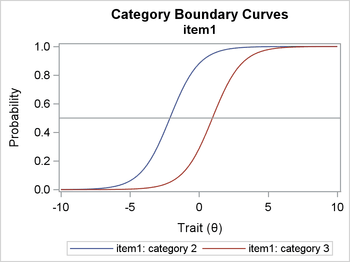### Plotting Option Response Functions

The following SAS statements create the macro %ORF, which plots the option response functions. The macro has one required argument, DATA=, which specifies the name of the output data set that you specify in the OUT= argument when you invoke the %PLOTS macro. The %ORF macro loops through the items and the categories for each item and uses PROC SGPLOT to generate an ORF plot for each item. It uses the global macro variables that are created by the %DIMENSIONS macro as the parameters for the loops and to create the titles for the plots.

```%macro orf(DATA=);
options nonotes;
title "Option Response Functions";
%do i=1 %to &n;
proc sgplot data=&data;
title2 "&&item&i";
%do j=1 %to &&dim&i;
series x=theta y=p&i&j;
%end;
yaxis label="Probability";
xaxis label="Trait ((*ESC*){unicode theta})";
refline .5 / axis=y;
run;
%end;
title;
%mend orf;
```

You invoke the macro by submitting the following statement (but supplying any data set name that you want for the input data set):

```%orf(data=plots)
```

Figure 2 displays the resulting ORF plots for the three items, which show the marginal probabilities of scoring in or selecting the kth category of item j over a range of values of .

Figure 2: ORF Plots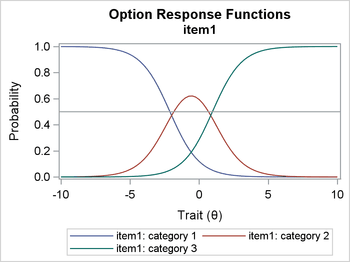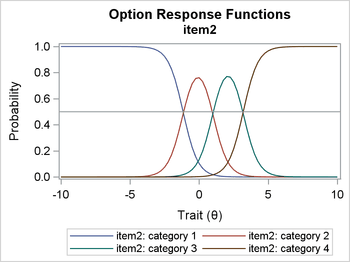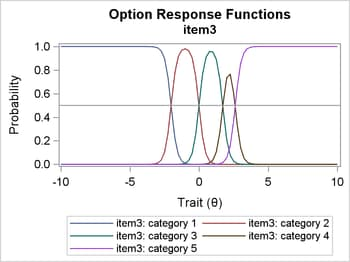## Plotting Option and Item Information Curves

The following SAS statements create the macro %IIC, which plots the option information curves and an item information curve for each item. The macro has one required argument, DATA=, which specifies the name of the output data set that you specify in the OUT= argument when you invoke the %PLOTS macro. The %IIC macro loops through the items and the categories for each item and uses PROC SGPLOT to generate a OIC plot for each option and an IIC for each item. It uses the global macro variables that are created by the %DIMENSIONS macro as the parameters for the loops and to create the titles for the plots.

```%macro iic(DATA=);
options nonotes;
title "Category & Item Information Curves";
%do i=1 %to &n;
proc sgplot data=&data;
title2 "&&item&i";
%do j=1 %to &&dim&i;
series x=theta y=ci&i&j;
%end;
series x=theta y=i&i;
yaxis label="Information";
xaxis label="Trait ((*ESC*){unicode theta})";
run;
%end;
title;
%mend iic;
```

You invoke the macro by submitting the following statement (but supplying any data set name that you want for the input data set):

```%iic(data=plots)
```

Figure 3 displays the resulting CIC and IIC plots for the three items. The CIC plots display the option information functions for each category of each item over a range of values of (OIC). The IIC plots display the sum of the option information functions for each item.

Figure 3: CIC and IIC Plots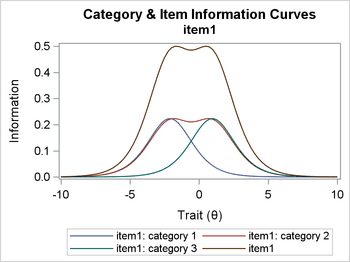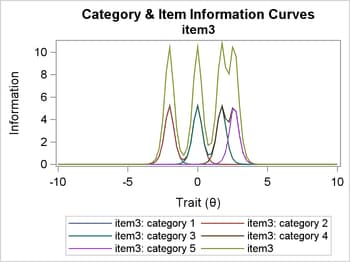## Plotting the Test Information Curve

The following SAS statements create the macro %TIC, which plots the test information curve. The macro has one required argument, DATA=, which specifies the name of the output data set that you specify in the OUT= argument when you invoke the %PLOTS macro. The %TIC macro does exactly what the manual version does; its only virtue is to eliminate the need to copy and paste the original manually generated program.

```%macro tic(DATA=);
options nonotes;
proc sgplot data=&data;
title "Test Information Curve";
series x=theta y=info;
yaxis label="Information";
xaxis label="Trait ((*ESC*){unicode theta})";
run;
title;
%mend tic;
```

You invoke the macro by submitting the following statement (but supplying any data set name that you want for the input data set):

```%tic(data=plots)
```

Figure 4 displays the resulting TIC plot, which displays the sum of all the item information functions.

Figure 4: Test Information Curve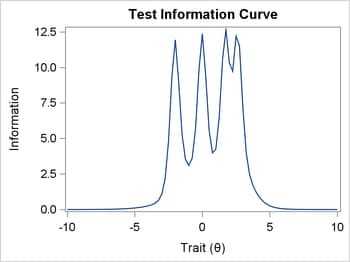## References

• De Ayala, R. J. (2009). The Theory and Practice of Item Response Theory. New York: Guilford Press.